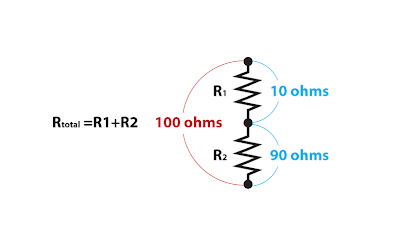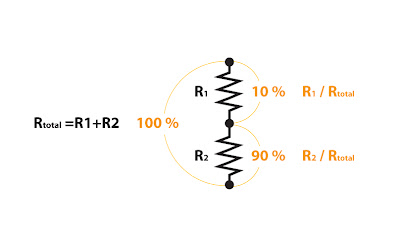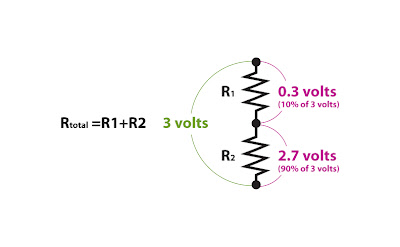## Friday, January 30, 2009

### What is a voltage divider? Pt1

You'll find voltage dividers in a whole host of places throughout your amp, your pedals and even in your guitar itself.

So what is it? And what does it do?

There are other applications, but one common use is as attenuators to reduce the voltage between stages. Once you understand the basic concept it's use in more complicated instances (like the divider formed with your plate load resistors) will be much easier to grasp.

The simplest sort is formed with two resistors hooked up in series:You'll see that the total resistance from the top of the first resistor (R1) to the bottom of the second (R2) is just the sum of the two resistors (Rtotal).

Rtotal makes up 100% of the total resistance of the circuit. The 10 ohm resistor R1 makes up one tenth of the total resistance - so .1 or 10%. R2 makes up the remainder, or 90%.So let's put a voltage across the two resistors, say 3 Volts:The full voltage, 3 volts, could be measured across the two resistors. If you add the voltages that appear across each of the resistors individually they must equal the total voltage of 3 volts. How much voltage appears across each resistor?

Take resistor R1. It makes up 10% of the total resistance of the circuit. Take 10% of the total voltage (that's 3 times .1) and you'll get the voltage drop across R1. That's .3 volts.

Do the same for R2 (3 times .9) and you'll get 2.7 volts.

Add those two and you'll get 3 volts. So things check out.

Now because we're looking at voltage not current we can change the resistances and get the same results as long as the ratio between R1 and R2 stays the same.

Here's the same circuit with R1=50 ohms and R2=450 ohms:When you do the math:

R1 divided by Rtotal = 500/50 = .1 or 10%
R2 divided by Rtotal =500/450 = .9 or 90%You can see this is the same as in the previous example.

So why does all this matter? Let's imagine the resistors in a more complete circuit:Here imagine that the input is coming from one amplifier stage the output is feeding the next stage. If we look again at how the voltages divide across the resistors:You'll see that with 3 volts of input you a .3 volt drop and the output voltage is reduced to 2.7 volts. Changing the values of the resistors can change the amount of drop. If you use a variable resistor (a potentiometer or "pot") you can vary the drop by turning the knob on the pot. This is exactly how the volume knobs on you guitar and amplifier work. I'll cover that in more detail in part 3.

#### 1 comment:Anonymous said...

I'm so happy to have found your site. You manage to explain things very clearly. Thanks so much!